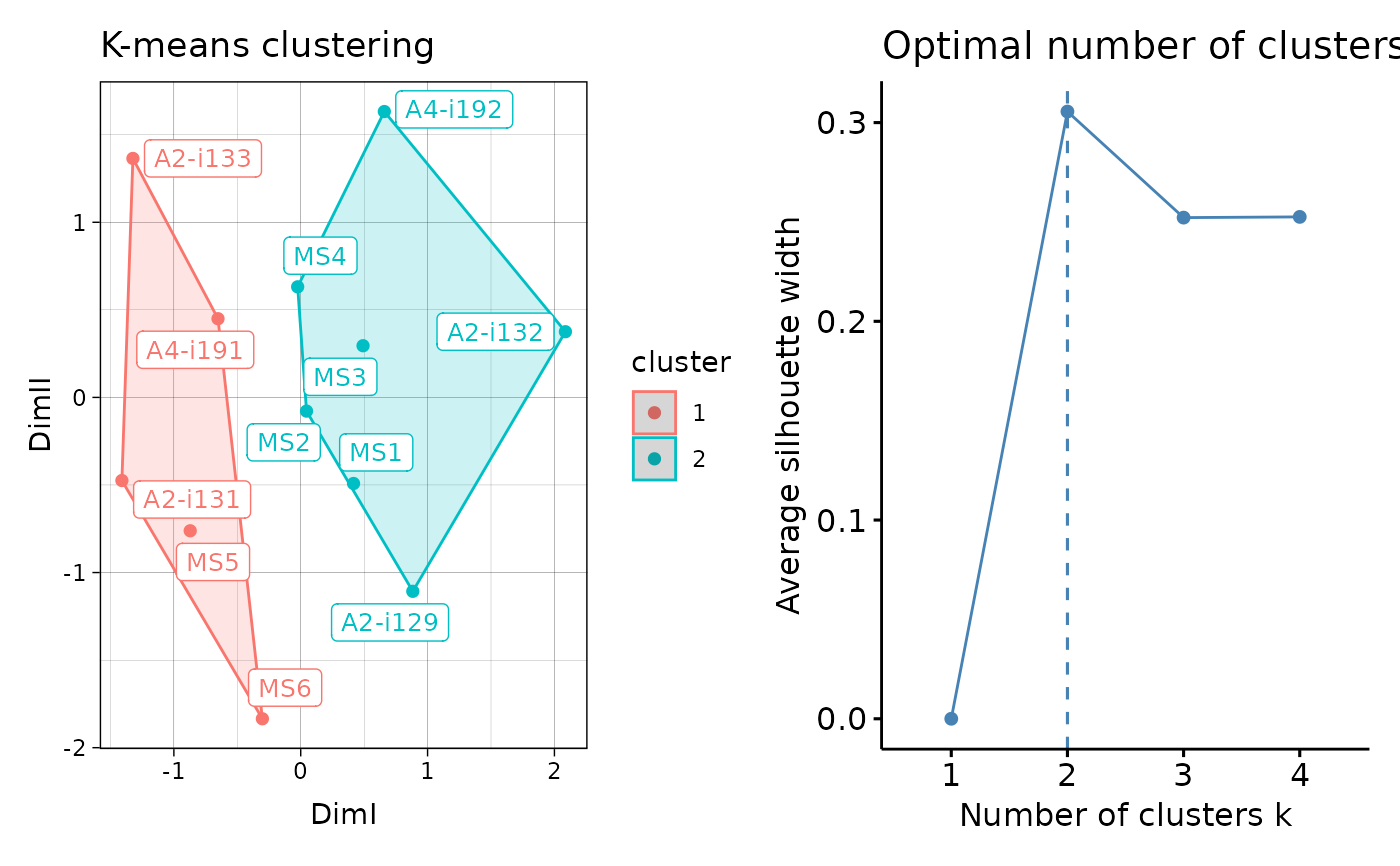Visualisation of the results of K-means and DBSCAN clustering. For hierarhical clustering visualisations see vis.immunr_hclust.

# S3 method for immunr_kmeans
vis(
.data,
.point = TRUE,
.text = TRUE,
.ellipse = TRUE,
.point.size = 2,
.text.size = 10,
.plot = c("clust", "best"),
...
)

## Arguments

.data

Clustering results from repOverlapAnalysis or geneUsageAnalysis.

.point

If TRUE then plot sample points. Passed to fviz_cluster.

.text

If TRUE then plot text labels. Passed to fviz_cluster.

.ellipse

If TRUE then plot ellipses around all samples. Passed to "ellipse" from fviz_cluster.

.point.size

Size of points, passed to "pointsize" from fviz_cluster.

.text.size

Size of text labels, passed to labelsize from fviz_cluster.

.plot

A character vector of length one or two specifying which plots to visualise. If "clust" then plot only the clustering. If "best" then plot the number of optimal clusters. If both then plot both.

...

Not used here.

## Value

Ggplot2 objects inside the pathwork container.

data(immdata)